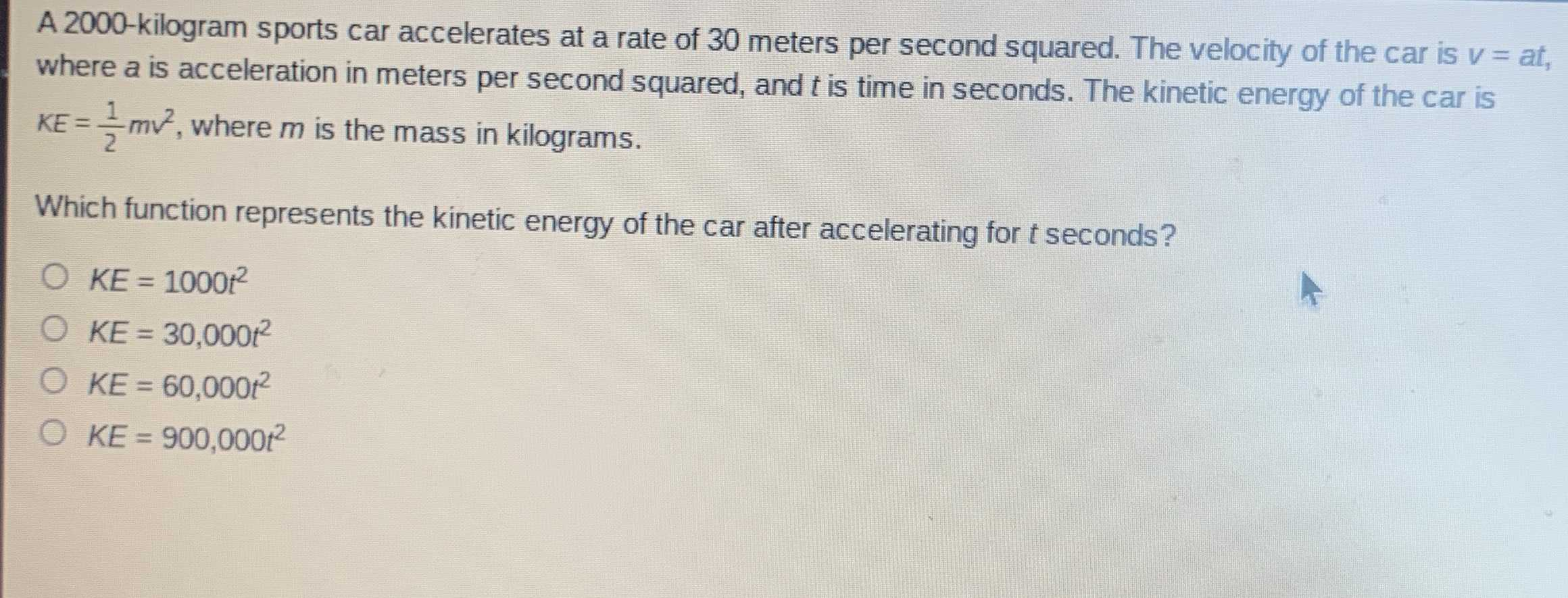### ¿Todavía tienes preguntas de matemáticas?

Pregunte a nuestros tutores expertos
Algebra
Pregunta$$2000$$ -kilogram sports car accelerates at a rate of $$30$$ meters per second squared. The velocity of the car is $$v =$$ at, where $$a$$ is acceleration in meters per second squared, and $$t$$ is time in seconds. The kinetic energy of the car is $$K E = \frac { 1 } { 2 } m v ^ { 2 }$$ , where $$m$$ is the mass in kilograms. Which function represents the kinetic energy of the car after accelerating for $$t$$ seconds?

$$K E = 1000 t ^ { 2 }$$

$$K E = 30,000 t ^ { 2 }$$

$$K E = 60,000 t ^ { 2 }$$

$$K E = 900,000 t ^ { 2 }$$